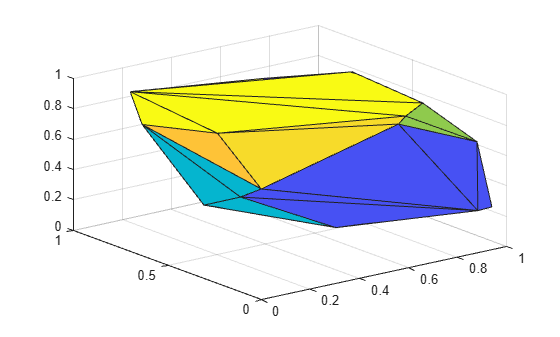# delaunayTriangulation

Delaunay triangulation in 2-D and 3-D

## Description

Use the `delaunayTriangulation` object to create a 2-D or 3-D Delaunay triangulation from a set of points. For 2-D data, you can also specify edge constraints.

You can perform a variety of topological and geometric queries on a `delaunayTriangulation`, including any `triangulation` query. For example, locate a facet that contains a specific point, find the vertices of the convex hull, or compute the Voronoi Diagram.

## Creation

To create a `delaunayTriangulation` object, use the `delaunayTriangulation` function with input arguments that define the triangulation's points and constrained edges.

### Syntax

``DT = delaunayTriangulation(P)``
``DT = delaunayTriangulation(P,C)``
``DT = delaunayTriangulation(x,y)``
``DT = delaunayTriangulation(x,y,C)``
``DT = delaunayTriangulation(x,y,z)``
``DT = delaunayTriangulation()``

### Description

example

````DT = delaunayTriangulation(P)` creates a Delaunay triangulation from the points in `P`. The matrix `P` has 2 or 3 columns, depending on whether your points are in 2-D or 3-D space.```
````DT = delaunayTriangulation(P,C)` specifies the edge constraints in the matrix `C` for the 2-D points in `P`. Each row of `C` defines the start and end vertex IDs of a constrained edge. Vertex IDs are the row numbers of the corresponding vertices in the property `DT.Points`.```
````DT = delaunayTriangulation(x,y)` creates a 2-D Delaunay triangulation from the point coordinates in the column vectors `x` and `y`.```
````DT = delaunayTriangulation(x,y,C)` specifies the edge constraints in a matrix `C`.```

example

````DT = delaunayTriangulation(x,y,z)` creates a 3-D Delaunay triangulation from the point coordinates in the column vectors `x`, `y`, and `z`. ```
````DT = delaunayTriangulation()` creates an empty Delaunay triangulation.```

### Input Arguments

expand all

Points, specified as a matrix whose columns are the x-coordinates, y-coordinates, and (possibly) `z`-coordinates of the triangulation points. The row numbers of `P` are the vertex IDs in the triangulation.

x-coordinates of triangulation points, specified as a column vector.

y-coordinates of triangulation points, specified as a column vector.

z-coordinates of triangulation points, specified as a column vector.

Vertex IDs of constrained edges, specified as a 2-column matrix. Each row of `C` corresponds to a constrained edge and contains two IDs:

• `C(j,1)` is the ID of the vertex at the start of an edge.

• `C(j,2)` is the ID of the vertex at end of the edge.

You can specify edge constraints for 2-D triangulations only.

## Properties

expand all

Points in the triangulation, represented as a matrix with the following characteristics:

• Each row in `DT.Points` contains the coordinates of a vertex.

• Each row number of `DT.Points` is a vertex ID.

Triangulation connectivity list, represented as a matrix with the following characteristics:

• Each element in `DT.ConnectivityList` is a vertex ID.

• Each row represents a triangle or tetrahedron in the triangulation.

• Each row number of `DT.ConnectivityList` is a triangle or tetrahedron ID.

Constrained edges, represented as a 2-column matrix of vertex IDs. Each row of `DT.Constraints` corresponds to a constrained edge and contains two IDs:

• `DT.Constraints(j,1)` is the ID of the vertex at the start of an edge.

• `DT.Constraints(j,2)` is the ID of the vertex at end of the edge.

`DT.Constraints` is an empty matrix when the triangulation has no constrained edges.

## Object Functions

 `convexHull` Convex hull of Delaunay triangulation `isInterior ` Query interior points of Delaunay triangulation `voronoiDiagram` Voronoi diagram of Delaunay triangulation `barycentricToCartesian` Convert coordinates from barycentric to Cartesian `cartesianToBarycentric` Convert coordinates from Cartesian to barycentric `circumcenter` Circumcenter of triangle or tetrahedron `edgeAttachments` Triangles or tetrahedra attached to specified edge `edges` Triangulation edges `faceNormal` Triangulation unit normal vectors `featureEdges` Handle sharp edges of triangulation `freeBoundary` Free boundary facets `incenter` Incenter of triangulation elements `isConnected` Test if two vertices are connected by an edge `nearestNeighbor` Closest vertex `neighbors` Triangle or tetrahedron neighbors `pointLocation` Triangle or tetrahedron enclosing point `size` Size of triangulation connectivity list `vertexAttachments` Triangles or tetrahedra attached to vertex `vertexNormal` Triangulation vertex normal

## Examples

collapse all

Create a 2-D `delaunayTriangulation` object for 30 random points.

```rng default; P = rand([30 2]); DT = delaunayTriangulation(P)```
```DT = delaunayTriangulation with properties: Points: [30x2 double] ConnectivityList: [48x3 double] Constraints: [] ```

Compute the center points of each triangle, and plot the triangulation with the center points.

```IC = incenter(DT); triplot(DT) hold on plot(IC(:,1),IC(:,2),'*r')```Create a 3-D `delaunayTriangulation` object for 30 random points.

```rng default; x = rand([30 1]); y = rand([30 1]); z = rand([30 1]); DT = delaunayTriangulation(x,y,z)```
```DT = delaunayTriangulation with properties: Points: [30x3 double] ConnectivityList: [102x4 double] Constraints: [] ```

Plot the triangulation.

`tetramesh(DT,'FaceAlpha',0.3);`Compute and plot the convex hull of the triangulation.

```[K,v] = convexHull(DT); trisurf(K,DT.Points(:,1),DT.Points(:,2),DT.Points(:,3))```expand all